# 简单线性回归(Simple Linear Regression)问题和举例

28 篇文章 0 订阅

0. 前提介绍：

0.1 集中趋势衡量
0.1.1 均值（平均数，平均值）（mean）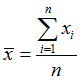{6, 2, 9, 1, 2}
(6 + 2 + 9 + 1 + 2) / 5 = 20 / 5 = 4
0.1.2中位数 （median）: 将数据中的各个数值按照大小顺序排列，居于中间位置的变量
0.1.2.1. 给数据排序：1， 2， 2， 6， 9
0.1.2.2. 找出位置处于中间的变量：2
当n为基数的时候：直接取位置处于中间的变量
当n为偶数的时候，取中间两个量的平均值
0.1.2 众数 （mode）：数据中出现次数最多的数
0.2
0.2.1. 离散程度衡量
0.2.1.1 方差（variance)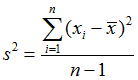{6, 2, 9, 1, 2}
(1) (6 - 4)^2 + (2 - 4) ^2 + (9 - 4)^2 + (1 - 4)^2 + (2 - 4)^2
= 4 + 4 + 25 + 9 + 4
= 46
(2) n - 1 = 5 - 1 = 4
(3) 46 / 4 = 11.5
0.2.1.2 标准差 (standard deviation)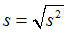s = sqrt(11.5) = 3.39

1. 介绍：回归(regression) Y变量为连续数值型(continuous numerical variable)
如：房价，人数，降雨量
分类(Classification): Y变量为类别型(categorical variable)
如：颜色类别，电脑品牌，有无信誉
2. 简单线性回归(Simple Linear Regression)
2.1 很多做决定过过程通常是根据两个或者多个变量之间的关系
2.3 回归分析(regression analysis)用来建立方程模拟两个或者多个变量之间如何关联
2.4 被预测的变量叫做：因变量(dependent variable), y, 输出(output)
2.5 被用来进行预测的变量叫做： 自变量(independent variable), x, 输入(input)
3. 简单线性回归介绍
3.1 简单线性回归包含一个自变量(x)和一个因变量(y)
3.2 以上两个变量的关系用一条直线来模拟
3.3 如果包含两个以上的自变量，则称作多元回归分析(multiple regression)
4. 简单线性回归模型
4.1 被用来描述因变量(y)和自变量(X)以及偏差(error)之间关系的方程叫做回归模型
4.2 简单线性回归的模型是: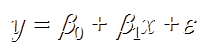5. 简单线性回归方程
E(y) = β 0 1
这个方程对应的图像是一条直线，称作回归线
其中， β 0 是回归线的截距
β 1 是回归线的斜率
E(y)是在一个给定x值下y的期望值（均值）

6. 正向线性关系：
7. 负向线性关系：
8. 无关系:
9. 估计的简单线性回归方程
ŷ=b 0 +b 1 x
这个方程叫做估计线性方程(estimated regression line)
其中， b 0 是估计线性方程的纵截距
b 1 是估计线性方程的斜率
是在自变量x等于一个给定值的时候，y的估计值

10. 线性回归分析流程：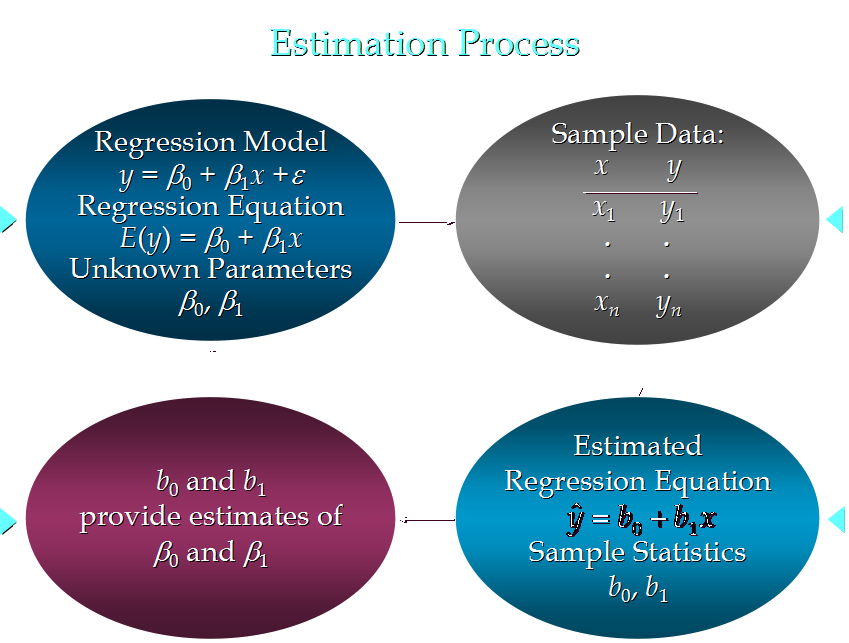11. 关于 偏差 ε的假定
11.1 是一个随机的变量，均值为0
11.2  ε的方差(variance)对于所有的自变量x是一样的
11.3  ε的值是独立的
11.4  ε满足正态分布
12.举例子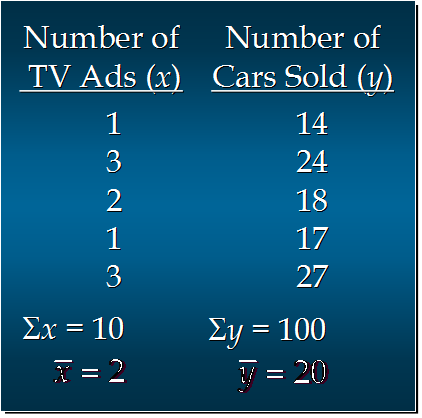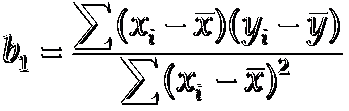= 6 + 4 + 0 + 3 + 7
= 20

= 1 + 1 + 0 + 1 + 1
4

b1 = 20/4  =5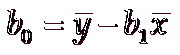b0 = 20 - 5*2 = 20 - 10 = 10

x_given = 6
Y_hat = 5*6 + 10 = 40

13.Python计算代码：
import numpy as np

def fitSLR(x, y):
n = len(x)
dinominator = 0
numerator = 0
for i in range(0, n):
numerator += (x[i] - np.mean(x))*(y[i] - np.mean(y))
dinominator += (x[i] - np.mean(x))**2
b1 = numerator/float(dinominator)
b0 = np.mean(y)/float(np.mean(x))
return b0, b1

def predict(x, b0, b1):
return b0 + x*b1

x = [1, 3, 2, 1, 3]
y = [14, 24, 18, 17, 27]

b0, b1 = fitSLR(x, y)

print "intercept:", b0, " slope:", b1

x_test = 6

y_test = predict(6, b0, b1)

print "y_test:", y_test


08-171034
05-0227901-31110
06-061699
03-07185
08-012212
01-18233
01-071万+
08-248380
08-17568
10-26425
12-04927
05-061万+
03-15275
05-092506
03-19345
09-04290
10-06477
09-20982点击重新获取扫码支付余额充值